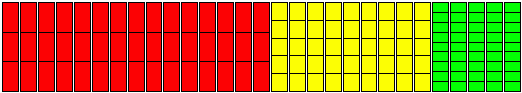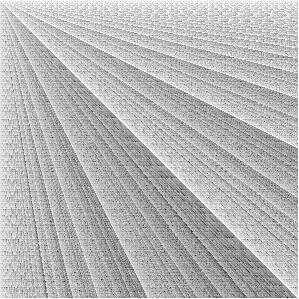## Egyptian Fractions

Ed Pegg Jr., June 21, 2004

Imagine overseeing a farm. Distribute 29 equal sacks of grain evenly to 45 people. There is only one sorting table, so you can't divide up more than one sack at a time. How can you assure a fair share for each person?

Consider this: 1/3 + 1/5 + 1/9 = 29/45. So, 15 of the sacks can be divided into 3 parts, 9 of them into 5 parts, and 5 of them into 9 parts. Then, each of the 45 people gets one of each type of part. In the diagram below, each person gets a red, yellow, and green part.Figure 1. Dividing 29 sacks equally between 45 people. Each person gets.

This problem dates back 4000 years. At the time, algebra and geometry didn't exist. No decimals. Whole numbers existed, but not fractions like 29/45. The ancient Egyptians would indicate a "part" number by a symbol that looked like a mouth. Thus, "part 7" would indicate 1/7. An Egyptian fraction would be something like part 3 + part 5 + part 9. In other words, 1/3 + 1/5 + 1/9 = 29/45. As seen above, this system was useful in dividing sacks of grain.

How smart were the ancient Egyptians? Try testing your skills against theirs. Find three different whole numbers, all less than 600, so that their reciprocals (the "parts") add up to 2/95. Consider 48 and 4560. As reciprocals, 1/48 + 1/4560 = 2/95. That doesn't solve the problem, since 4560 exceeds 600. Use a computer, or anything you like. The solution is below.

In 2000 BC, a table of these solutions was recorded for posterity. Later, in 1600 BC, a scribe by the name Ahmes found the document, and recorded the "ancient" results. He also wrote down some math problems to be solved just for fun, which makes him the earlier known recreational mathematician. Much later, in 1858 AD, Ahmes' papyrus scroll was bought by Henry Rhind in Thebes. Eventually, the scroll went to the British Museum. The first two columns of the table below are the numbers scribed by Ahmes.

 Fraction Ahmes (Rhind) Papyrus, 1/a + 1/b as a + b 2/p = 1/A + (2A - p)/Ap, with A = (p+1)/2 Other representations of interest 2/3 2 + 6 2 + 6 2/5 3 + 15 3 + 15 2/7 4 + 28 4 + 28 6 + 14 + 21 2/9 6 + 18 5 + 45 2/11 6 + 66 6 + 66 2/13 8 + 52 + 104 7 + 91 10 + 26 + 65 2/15 10 + 30 8 + 120 12 + 20 2/17 12 + 51 + 68 9 + 153 2/19 12 + 76 + 114 10 + 190 2/21 14 + 42 11 + 231 15 + 35 2/23 12 + 276 12 + 276 2/25 15 + 75 13 + 325 2/27 18 + 54 14 + 378 2/29 24 + 58 + 174 + 232 15 + 435 2/31 20 + 124 + 155 16 + 496 2/33 22 + 66 17 + 561 2/35 30 + 42 18 + 630 2/37 24 + 111 + 296 19 + 703 2/39 26 + 78 20 + 780 52 + 60 + 65 2/41 24 + 246 + 328 21 + 861 2/43 42 + 86 + 129 + 301 22 + 946 2/45 30 + 90 23 + 1035 36 + 60 2/47 30 + 141 + 470 24 + 1128 2/49 28 + 196 25 + 1225 42 + 98 + 147 2/51 34 + 102 26 + 1326 2/53 30 + 318 + 795 27 + 1431 2/55 30 + 330 28 + 1540 40 + 88 2/57 38 + 114 29 + 1653 2/59 36 + 236 + 531 30 + 1770 2/61 40 + 244 + 488 + 610 31 + 1891 2/63 42 + 126 32 + 2016 56 + 72 2/65 39 + 195 33 + 2145 45 + 117 2/67 40 + 335 + 536 34 + 2278 2/69 46 + 138 35 + 2415 2/71 40 + 568 + 710 36 + 2556 2/73 60 + 219 + 292 + 365 37 + 2701 2/75 50 + 150 38 + 2850 60 + 100 2/77 44 + 308 39 + 3003 63 + 99 2/79 60 + 237 + 316 + 790 40 + 3160 2/81 54 + 162 41 + 3321 2/83 60 + 332 + 415 + 498 42 + 3486 2/85 51 + 255 43 + 3655 55 + 187 2/87 58 + 174 44 + 3828 2/89 60 + 356 + 534 + 890 45 + 4005 2/91 70 + 130 46 + 4186 2/93 62 + 186 47 + 4371 2/95 60 + 380 + 570 48 + 4560 2/97 56 + 679 + 776 49 + 4753 2/99 66 + 198 50 + 4950 90 + 110 2/101 101 + 202 + 303 + 606 51 + 5151
Figure 2. The Ahmes 2/n table. (1600 BC)

In answer to the earlier question, 2/95 = 1/60 + 1/380 + 1/570. The Ahmes table has many "best" solutions, with either the lowest largest denominator, or other optimizations. How did Ahmes do it?

One method, suggested by Akhmim P. Eves, uses n/pq = 1/(p(p + q)/n) + 1/(q(p + q)/n). For example:
2/35, p = 5, q = 7, or 2/(5 7) = 1/(5(5+7)/2) + 1/(7(5+7)/2) = 1/30 + 1/42
2/91, p = 7, q = 13, or 2/(7 13) = 1/(7(7+13)/2) + 1/(13(7+13)/2) = 1/70 + 1/130

Note the solution for 2/101, namely 2/p = 1/p + 1/2p + 1/3p + 1/6p. Ahmes knew of some kind of proto-number theory. Every composite entry in the table is based on a decomposition of n into its prime factors, so he knew about prime numbers. Notice, as well, how often 60 pops up -- under 2/89, for example. In solutions of this type, "part 60" is a very useful number.

Milo Gardner has written a paper on the Ahmes 2/n table. Based on the sophisticated results contained by it, Gardner argues that Ahmes and his associates knew more mathematics than they are given credit for. Myself, I had a computer at my disposal, and ran a number of brute-force searches to try to beat Ahmes.

A computer check reveals that Ahmes found the best solutions most of the time. I used the notebook Ten Algorithms for Egyptian Fractions by David Eppstein in this study (his page on Egyptian Fractions is also excellent).

Here is an interesting sequence of fractions that would likely would have fascinated Ahmes: 1/2, 2/3, 4/5, 8/11, 14/17, 19/23, 24/29, 49/59, 65/71, 76/83, 61/157, 183/191, 260/269, 289/299. 8/11 = 1/2 + 1/6 + 1/21 + 1/77. This is the simplest Egyptian fraction that requires 4 parts. 14/17 = 1/2 + 1/4 + 1/20 + 1/55 + 1/187 requires 5 parts. 289/299 is the simplest fraction that requires 14 parts. One might think that this sort of thing was well known, but it isn't. The below image gives the number of parts needed for 2/2 (upper left) to 299/299 (lower right), with darker pixels for the fractions that require more parts. Only 289/299 has the darkest pixel, here, with 14 parts.Figure 2. The number of Parts required for various fractions.

If the image was extended, would anything in the 4/n column ever require 4 parts? No-one knows -- it's a long unsolved question. So far, a three part solution has been found for every given 4/n fraction, but no proof has been found that a three part solutions always exists. What is the simplest fraction that requires 15 parts, 16 parts, and beyond? Just how much math did Ahmes know? I close with a quote by him.

Accurate reckoning: the entrance into knowledge of all existing things and all obscure secrets. -- Ahmes, 1600 BC

Mathematica Code:

Code used for this column can be found in the Mathematica Information Center, at the Ten Algorithms for Egyptian Fractions entry, by David Eppstein.

References:

David Eppstein, Ten Algorithms for Egyptian Fractions, Mathematica in Education and Research, 1995, p5-15.

Milo Gardner, "The Egyptian Mathematical Leather Scroll," http://www.math.buffalo.edu/mad/Ancient-Africa/gardner.EMLRL.160202.doc.

Milo Gardner, "The Best Egyptian Fractions," http://www.math.buffalo.edu/mad/Ancient-Africa/best-egyptian-fraction.html.

J J O'Connor and E F Robertson, "Egyptian numerals," http://www-gap.dcs.st-and.ac.uk/~history/HistTopics/Egyptian_numerals.html.